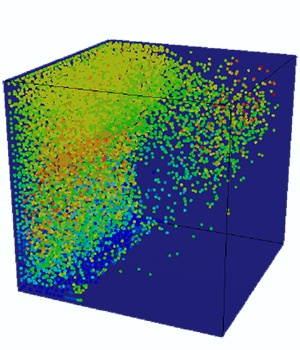# Abaqus Tutorial 23:Sloshing in a tank modeled using SPH as an example

This tutorial gives a basic introduction to SPH modeling in Abaqus CAE. The tutorial will take you through a basic model of g forces acting on a fluid in a typical tank.

When you complete this tutorial, you will be able to:

• Create the right materials and properties for water and aluminum.
• Mesh an aluminum tank with a water block.
• Create the best results from the model by post processing.
• Change variables plotted on the geometry.

Get your FREE Abaqus tutorial now!